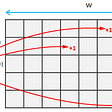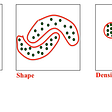# Discrete Probability Distribution : Part 1

## What are discrete random variables and discrete probability distribution?

In probability and statistics, a random variable is a quantitative variable whose value depends on the outcomes of a random phenomenon. For example, let us toss a coin 3 times and let X represents the number of tails that appear in that 3 tosses. Then X can take any one of the following values : 0, 1, 2, 3. Here X will be the random variable as it represents the chances or outcomes of tossing the coin. We don’t know the results until the coin is tossed and calculate the number of tails. Here the variable X will be discrete. This means it can take only exact values.

Discrete random variables can take on a finite or countable number of possible values whereas in the case of continuous random variables, they can take any or infinite number of values in an interval.

We can also list down the probabilities of occurring of all the possible outcomes for tossing the coin 3 times. The possible outcomes are : HHH, THH, HTH, HHT, HTT, THT, TTH, and TTT. Here H represents head and T represents the tail.

The above picture represents the probability distribution of random variable X. It is a listing of all possible values of X and their probabilities of occurring, p(x). If we repeat a random experiment many times and plot probabilities of each possible outcome, then we will get probability distribution.

A discrete probability distribution must satisfy the following two conditions :

1. 0≤ p(x) ≤ 1, for all values of x.

2. ∑ p(x) = 1

In our example, ∑ p(x) = 0.125+0.375 +0.375+ 0.125 = 1

# The expected value, variance, and standard deviation of discrete probability distribution :

The expected value or expectation of a random variable is the long-term theoretical mean of the random variable. We can calculate the expected value, E(X) by multiplying each value of X with their respective probability of occurring and then sum up all of them.

# E(X=x) = ∑ x. p(x)

For example, say one of your friends offers you to play a game. A dice will be rolled and the following are the rules for the game :

1. If it comes up 1, you have to give him Rs.1.
2. If it comes up 2, he will give you Rs. 2.
3. If it comes up 3, you have to give him Rs.3.
4. If it comes up 4, he will give you Rs. 4.
5. If it comes up 5, you have to give him Rs.5.
6. If it comes up 6, he will give you Rs. 6.

Would you play the game? You will play the game only if it is profitable. To know this we have to calculate expected winning or loss from the game. The probability distribution for the game will be as follows :

E(X) = (-1 x 1/6) +(2 x 1/6) +(-3 x 1/6) + (4 x 1/6) + (-5 x 1/6) +(6 x 1/6)

= 3/6

= Rs. 0.5

Since the expected value is Rs. 0.5, it will be a profitable game. In other words, you can expect to win Rs. 0.5 for each game. If you play the game 1000 times, then the amount you should expect to win will be (0.5 x1000) = Rs. 500. The same game was simulated 1000 times and the following two pictures are the results of the simulation which are showing total winning and average winning per game after playing the game 1000 times.

The above pictures clearly show that after playing the game 1000 times, the total winning is nearly equal to Rs.500 and average winning per game is near Rs.0.5. This is what we expected. The long term mean of the winnings is matching with the expected value and the total winning is also close to the expected Rs. 500. Initially, both total winning and average winning per game fluctuate a lot. But in long term, these tend to deviate less from the expectation.

The expected value gives us the typical or average value of a variable. But it does not tell anything about how the values are spread out. Here comes the variance which will tell us about the data spread.

# Var (X) = E(x-µ)² = ∑(x-µ)².p(x)

Let us take the above example of game again. Here, E(X) or µ is 0.5.

Hence, Var(X) = (-1- 0.5)² x 1/6 + (2 - 0.5)² x 1/6 + (-3- 0.5)² x 1/6 + (4 - 0.5)² x 1/6 + (-5- 0.5)² x 1/6 + (6 - 0.5)² x 1/6 = 14.92

Similarly, we can also calculate standard deviation according to following :

# Std. Dev = √Variance

Measures of variability and spread | by Dhrubjun | Nerd For Tech | Sep, 2021 | Medium

So, for the above example, standard deviation will be √14.92 = 3.86. That means on average, our winning per game will be 3.86 away from the expectation of 0.5.

That’s all for today. In next part, we will learn about different discrete probability distributions. Till then keep smiling. 😀

--

--

--

## More from Nerd For Tech

NFT is an Educational Media House. Our mission is to bring the invaluable knowledge and experiences of experts from all over the world to the novice. To know more about us, visit https://www.nerdfortech.org/.

## Turbocharging Derivatives — Variance, Convexity, and Everything in Between## Avast Ye!## Check if a Binary Tree is a Subtree of another Binary Tree## But what is a quantum phase factor?## Approximate Computing## Dhrubjun

Machine learning/Data Science enthusiast

## The Elements of Statistical Learning 2.4## Continuous Random Variable## Understanding Working Of Logistic Regression## Hierarchical Clustering